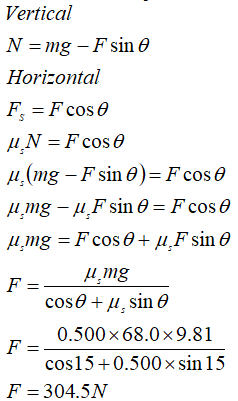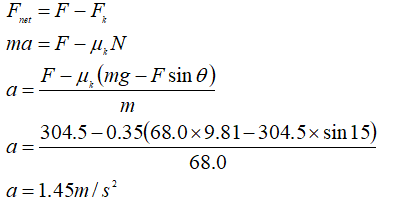# A 68.0 kg crate is dragged across a floor to the right by pulling on a rope attached to the crate and inclined 15.0° above the horizontal. (a) If the coefficient of static friction is 0.500, what minimum force magnitude is required from the rope to start the crate moving? (b) If the coefficient of kinetic friction is 0.350, what is the magnitude of the initial acceleration of the crate? Make a free-body diagram for part (b). Please use the blue vector to specify forces and the pink vector for acceleration. Make sure to label each vector with none, mg, fk, a, FN or T.

Question
153 views

A 68.0 kg crate is dragged across a floor to the right by pulling on a rope attached to the crate and inclined 15.0° above the horizontal. (a) If the coefficient of static friction is 0.500, what minimum force magnitude is required from the rope to start the crate moving? (b) If the coefficient of kinetic friction is 0.350, what is the magnitude of the initial acceleration of the crate?

Make a free-body diagram for part (b). Please use the blue vector to specify forces and the pink vector for acceleration.

Make sure to label each vector with none, mg, fk, a, FN or T.

check_circle

Step 1

Given

Mass m = 68.0 kg

Angle θ = 15.0o

Coefficient of static friction μs = 0.500

Coefficient of kinetic friction μk =0.350

Step 2

Solution

Minimum force required to move the crate

Since the crate is in equilibrium in vertical direction and horizontal directionStep 3

Initial acceleration...

### Want to see the full answer?

See Solution

#### Want to see this answer and more?

Solutions are written by subject experts who are available 24/7. Questions are typically answered within 1 hour.*

See Solution
*Response times may vary by subject and question.
Tagged in